# Bloch Sphere

## Single-qubit State

Unlike classical bits, qubits can be in both the computational basis vector $$\left|0\right>$$ state and the $$\left|1\right>$$ state, usually expressed as

$\left|\psi\right> = a\left|0\right> + b\left|1\right>$

Here $$a$$ and $$b$$ are complex numbers. Due to the normalization condition of the quantum state $$\left<\psi\middle|\psi\right> = 1$$, therefore

$\left|a\right|^2 + \left|b\right|^2 = 1$

For a two-dimensional Hilbert space, we can make the following mapping of the computational basis vector

$\begin{split}\left|0\right> = \begin{pmatrix} 1 \\ 0 \end{pmatrix}, \left|1\right> = \begin{pmatrix} 0 \\ 1 \end{pmatrix}\end{split}$

Thus, any single-qubit state can be expressed as

$\begin{split}\left|\psi\right> = \begin{pmatrix} a \\ b \end{pmatrix}\end{split}$

In the general case, we do not care about the global phase, so we can assume $$a=\cos\left(\theta/2\right), b=e^{i\phi}\sin\left(\theta/2\right)$$

$\left|\psi\right> = \cos\left(\theta/2\right) \left|0\right> + e^{i\phi}\sin\left(\theta/2\right)\left|1\right>$

Here we might as well represent that arbitrary quantum state in the unit ball, as follows, taking $$\theta$$ and $$\phi$$ as the elevation and azimuth angles, respectively.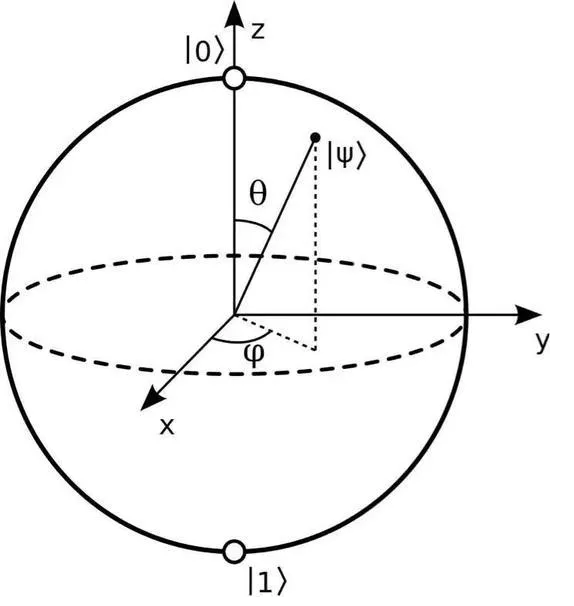Next we will show how to demonstrate a single-qubit state in MindSpore Quantum and the evolution of the single-qubit state in the form of an animation.

## Building Quantum Circuit

From the above bloch sphere, we can control the elevation angle $$\theta$$ by the revolving gate RX and the azimuth angle $$\phi$$ by RZ. Therefore, we can build the following quantum circuit.

:

circ = Circuit()               # Build circuit for preparing an arbitrary single-qubit quantum state
circ += RX('theta').on(0)      # Control elevation via RX gate
circ += RZ('phi').on(0)        # Control azimuth via RZ gate
circ.svg()

:Here we might as well take $$\theta=\pi/4, \phi=\pi/4$$ and calculate the corresponding quantum state.

:

import numpy as np

state1 = circ.get_qs(pr={'theta': np.pi/4, 'phi': np.pi/4})
print(state1)

[0.85355339-0.35355339j 0.14644661-0.35355339j]


## Displaying the Quantum State

In MindSpore Quantum, BlochScene is the module used to display the Bloch sphere. We can add as many single-qubit states as we want to the BlochScene and also animate the evolution of the single-qubit quantum states.

:

scene = BlochScene()                       # Create Bloch drawing scene
fig, ax = scene.create_scene()             # Initialize the scene
state_obj1 = scene.add_state(ax, state1)   # Add a quantum state to the scene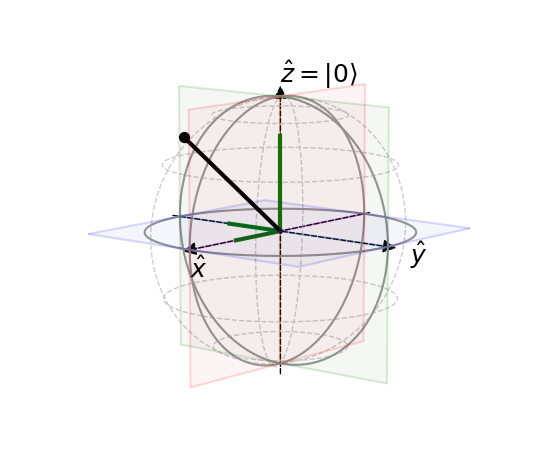In addition, we can also show the Bloch sphere in dark mode, as follows.

:

scene = BlochScene('dark')                       # Create Bloch drawing scene
fig, ax = scene.create_scene()                   # Initialize the scene
state_obj1 = scene.add_state(ax, state1)         # Add a quantum state to the scene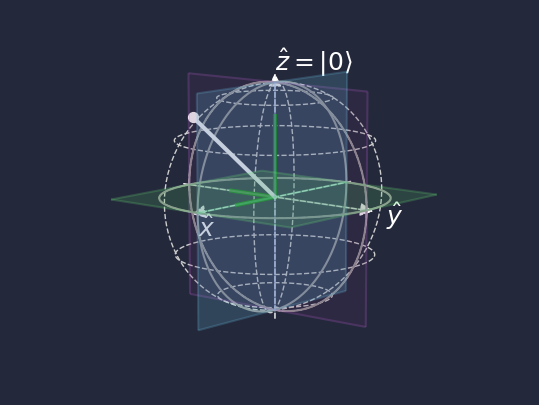## Displaying the Evolution of the Quantum State

We can also create animations in the Bloch scene when the quantum state is a time-dependent quantum state. Here we may assume that the elevation $$\theta$$ and azimuthal $$\phi$$ are time-dependent and we can obtain the quantum states for all time.

:

t = np.linspace(0, 10, 500)
all_theta = 4 * np.sin(2 * t)
all_phi = 5 * np.cos(3 * t)
states = []
for theta, phi in zip(all_theta, all_phi):
states.append(circ.get_qs(pr={'theta': theta, 'phi': phi}))
states = np.array(states)


In the following, we create a dark Bloch scene and initialize the scene with the first one of the evolved quantum states.

:

scene = BlochScene('dark')                          # Create Bloch drawing scene
fig, ax = scene.create_scene()                      # Initialize the scene
state_obj = scene.add_state(ax, states)          # Add a quantum state to the scene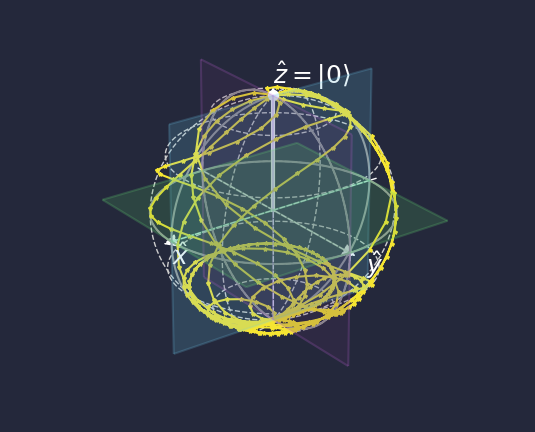To be able to display dynamically the evolution of quantum states, we create an animated object from the Bloch scene.

:

anim = scene.animation(fig, ax, state_obj, states)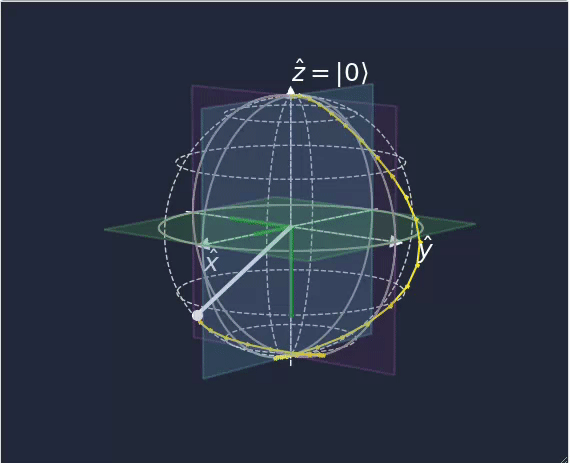From this, we can see that the quantum state of a single qubit has moved in the Bloch sphere.

:

from mindquantum.utils.show_info import InfoTable

InfoTable('mindquantum', 'scipy', 'numpy')

:

Software Version
mindquantum0.9.0
scipy1.10.1
numpy1.23.5
System Info
Python3.8.17
OSWindows AMD64
Memory8.39 GB